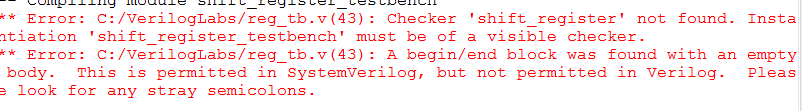Intel® Quartus® Prime Software
Intel® Quartus® Prime Design Software, Design Entry, Synthesis, Simulation, Verification, Timing Analysis, System Design (Platform Designer, formerly Qsys)
16336 DiscussionsNovice
1,211 Views

Hey i wrote this code in Verilog.

This is the Code:

``````module shift_register(write,s_in,clk,d,s_out,p);

input write,clk;

input [3:0] d;
input [11:0] s_in;

output reg s_out;
output reg [3:0] p;

wire mux_1,mux_2,mux_3,mux_4,dff_1,dff_2,dff_3,dff_4; // 8 wires

mux2_1 mux1(.s(s_in),.d(d),.w(write),.z(mux_1)); // mux1

D_FlipFlop dff1(.d(mux_1),.clk(clock),.q(dff_1)); // DFlipFlop1
always@(posedge clk) begin
p<=dff_1;
end
mux2_1 mux2(.s(dff_1),.d(d),.w(write),.z(mux_2));// mux2

D_FlipFlop dff2(.d(mux_2),.clk(clock),.q(dff_2));  // DFlipFlop2
always@(posedge clk) begin
p<=dff_2;
end
mux2_1 mux3(.s(dff_2),.d(d),.w(write),.z(mux_3));// mux3

D_FlipFlop dff3(.d(mux_3),.clk(clock),.q(dff_3));  // DFlipFlop3
always@(posedge clk) begin
p<=dff_3;
end
mux2_1 mux4(.s(dff_3),.d(d),.w(write),.z(mux_4));// mux4

D_FlipFlop dff4(.d(mux_4),.clk(clock),.q(dff_4));  // DFlipFlop4
always@(posedge clk) begin
p<=dff_4;

s_out<=dff_4;
end
endmodule``````

and this is the Testbench:

``````module shift_register_testbench();
wire write,clk;

input [3:0] d;
input [11:0] s_in;

output reg s_out;
output reg [3:0] p;

initial
begin
d[3:0]  = 1'b1;
write = 1'b0;

s_in = 1'b1;
#10;
s_in = 1'b0;
#10;
s_in = 1'b0;
#10;
s_in = 1'b1;
#10;
s_in = 1'b0;
#10;
s_in = 1'b0;
write = 1'b1;
#10;
s_in = 1'b1;
write = 1'b0;
#10;
s_in = 1'b0;
#10;
s_in = 1'b1;
#10;
s_in = 1'b1;
#10;
s_in = 1'b1;
#10;
s_in = 1'b0;
#10;

shift_register SRTB(.write(write),.s_in(s_in[11:0]),.clk(clk),.d(d[3:0]),.s_out(s_out),.p(p[3:0]));

end
endmodule

``````

And when I`m try to run the testbench this problem appear:how can i fix it?THNX!

3 RepliesHonored Contributor III
1,196 Views

Where is your shift register HDL file located?  Is it in the same directory as the testbench?  I think the issue is ModelSim is simply not seeing the file.  Make sure you "Change Directory" in ModelSim to point to the location of the code.

#iwork4intelModerator
1,192 Views

Hi Naor,

Make sure your HDL and testbench in same directory when you simulating the design. The directory in Modelsim is same as the path where your hdl and tb files.

Thanks,

RegardsNovice
1,172 Views

Yes i fixed it. Thanks. Close case please.